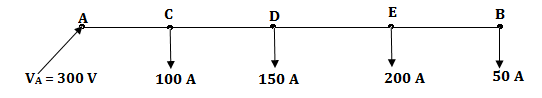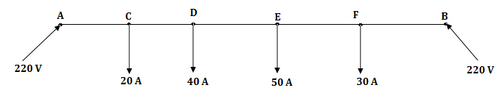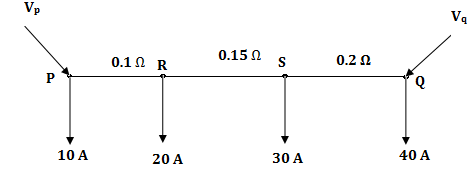# DC Distribution Calculations of Electrical Power - MCQs with Answers

## DC Distribution Calculations of Electrical Power - MCQs with Answers

Q1. What will be the pd at point B if a pd of 300 V is maintained at a point A, for a 2 wire dc distributor cable AB if 2 km long and supplies loads of 100A, 150A, 200A, and 50A suited at 500m, 1000m, 1600m, and 2000m from the feeding point A. Each conductor has a resistance of 0.01 Ω per 1000 m.a. 288 V
b. 287.6 V
c. 295.36 V
d. 291.2 V

ANSWER: b. 287.6 V

Q2. What is the loss factor in a distribution system?

a. 0.3 * load factor + 0.7 (load factor)2
b. 0.5 * (load factor)
d. 0.7 * load factor + 0.3 (load factor)2

ANSWER: a. 0.3 * load factor + 0.7 (load factor)2

Q3. A 2 wire dc street mains AB, 600 m long is fed from both ends at 220 V. Loads of 20 A, 40 A, 50 A and 30 A are tapped at distances 100m, 250m, 400m and 500m from the end A. If the area of cross section of distributor is 1 cm2, what will be the minimum consumer voltage? If ? = 1.7 * 10-6 Ω cm.a. 220 V
b. 215.69 V
c. 218.36 V
d. 222.58 V

ANSWER: b. 215.69 V

Q4. A distribution system is shown in figure with load currents as marked. The two ends of the feeder are fed by voltage sources such that Vp = Vq = 3 V, the value of the voltage Vp for a minimum voltage of 220 V at any point along the feeder isa. 225.89 V
b. 222.89 V
c. 220.0 V
d. 228.58 V

ANSWER: a. 225.89 V

Q5. A uniformly loaded dc distributor is fed at both ends with equal voltages. In comparison to a similar distributor fed at one end only, what will be the maximum voltage drop?

a. One fourth
b. Half
c. One third
d. One sixth

ANSWER: a. One fourth

Q6. A uniformly loaded dc distributor is fed at both ends with equal voltages. In comparison to a similar distributor fed at one end only, what will be the drop at the midpoint be?

a. One fourth
b. Half
c. One third
d. One sixth

ANSWER: c. One third

Q7. Installation of capacitors at suitable locations and of optimum size in a distribution system results in

i. Improved voltage regulation.
ii. Reduction in distribution power losses.
iii. Reduction of kVA rating of distribution transformers.

Which among the following is/are the correct codes?

a. i only
b. i and ii only
c. all of these
d. iii only